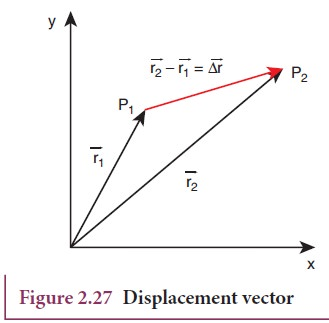Home | | Physics 11th std | Displacement Vector in Cartesian Coordinate System

# Displacement Vector in Cartesian Coordinate System

In terms of position vector, the displacement vector is given as follows

Displacement Vector in Cartesian Coordinate System

In terms of position vector, the displacement vector is given as follows. Let us consider a particle moving from a point P1 having position vectorThis displacement is also shown in Figure 2.27.## Solved Example Problems for Displacement Vector in Cartesian Coordinate System

### Example 2.17

Calculate the displacement vector for a particle moving from a point P to Q as shown below. Calculate the magnitude of displacement.### SolutionTags : with Solved Example Problems , 11th Physics : UNIT 2 : Kinematics
Study Material, Lecturing Notes, Assignment, Reference, Wiki description explanation, brief detail
11th Physics : UNIT 2 : Kinematics : Displacement Vector in Cartesian Coordinate System | with Solved Example Problems